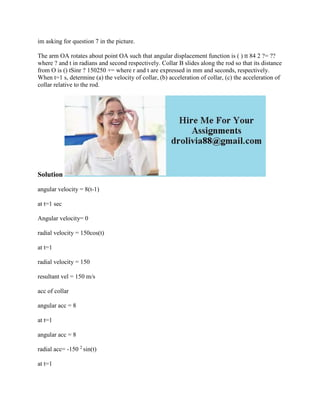Seu SlideShare está sendo baixado. ×

# im asking for question 7 in the picture- The arm OA rotates about poin.docx

Anúncio
Anúncio
Anúncio
Anúncio
Anúncio
Anúncio
Anúncio
Anúncio
Anúncio
Anúncio
AnúncioCarregando em…3
×

1 de 2 Anúncio

# im asking for question 7 in the picture- The arm OA rotates about poin.docx

im asking for question 7 in the picture.
The arm OA rotates about point OA such that angular displacement function is ( ) tt 84 2 ?= ?? where ? and t in radians and second respectively. Collar B slides along the rod so that its distance from O is () tSinr ? 150250 += where r and t are expressed in mm and seconds, respectively. When t=1 s, determine (a) the velocity of collar, (b) acceleration of collar, (c) the acceleration of collar relative to the rod.
Solution
angular velocity = 8(t-1)
at t=1 sec
Angular velocity= 0
at t=1
resultant vel = 150 m/s
acc of collar
angular acc = 8
at t=1
angular acc = 8
at t=1
resultent acc 8
relative acc= 8red/sec 2
.

im asking for question 7 in the picture.
The arm OA rotates about point OA such that angular displacement function is ( ) tt 84 2 ?= ?? where ? and t in radians and second respectively. Collar B slides along the rod so that its distance from O is () tSinr ? 150250 += where r and t are expressed in mm and seconds, respectively. When t=1 s, determine (a) the velocity of collar, (b) acceleration of collar, (c) the acceleration of collar relative to the rod.
Solution
angular velocity = 8(t-1)
at t=1 sec
Angular velocity= 0
at t=1
resultant vel = 150 m/s
acc of collar
angular acc = 8
at t=1
angular acc = 8